# The Convergence Rate in Precise Asymptotics for Series of Large Deviations

## Authors

• Юлія Олександрівна Грегуль NTUU KPI, Ukraine

## Keywords:

Precise asymptotics, Convergence rate, Series of large deviations

## Abstract

Background. In the paper the sequence of independent identically distributed random variables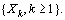is considered. We are interested in conditions for the convergence of series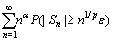for different values of parameters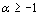,and any. Such series appears while studying complete convergence as well as investigating various problems on large deviations in limit theorems of probability theory. The new approach to study such series is proposed by C. Heyde, who showed that if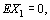then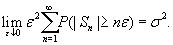Further, this result was extended by O. Klesov, and finally generalized by A. Gut, J. Steinebach and J. Hi for the serieswith, and.

Objective. We consider the seriesfor one-side deviations. The main purpose of the paper is to study precise asymptotics of functionwhileMethods. The methods used to prove the main result is as follows: first we find the asymptoticsfor the partial case, i.e. we assume that random variables are Gaussian random variables, further we extend obtained result to the general case by means of estimations of rate of convergence in the Central limit theorem.

Results. In the paper the precise asymptotics of serieswhilefor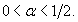is obtained. Strict assumptions imposed on parameter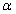are connected with the application of Nagaev inequality on the rate of convergence in the Central limit theorem.

Conclusions. The asymptoticsought to be considered for other values ofas well. This requires though new techniques since the use of Nagaev inequality leads to some restrictions upon.  Since seriesconverges (under some moment conditions) for any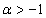then further investigations may concern the behavior of this series for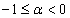and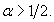## Author Biography

### Юлія Олександрівна Грегуль, NTUU KPI

Yuliya O. Gregul.
Assistant at the Department of Mathematical Analysis and Probability Theory

## References

P.L. Hsu and H. Robbins, “Complete convergence and the law of large numbers”, Proc. Nat. Acad. Sci.USA, vol. 33, pp. 25–31, 1947.

P. Erdös, “On a theorem of Hsu and Robbins”, Ann. Math. Statist., vol. 20, pp. 287–291, 1949.

F. Spitzer, “A combinatorial lemma and its application to probability theory”, Trans. Amer. Math. Soc., vol. 82, pp. 323–339, 1956.

M. Katz, “The probability in the tail of distribution”, Ann. Math. Statist., vol. 34, pp. 312–318, 1963.

L.E. Baum, M. Katz, “Convergence rates in the law of large numbers”, Trans. Amer. Math. Soc., vol. 120, pp. 108–123, 1965.

C.C. Heyde, “A supplement to the strong law of large numbers”, J. Appl. Probab., vol. 12, pp. 903–907, 1975.

O.I. Klesov, “On the convergence rate in a theorem of Heyde”, Theory Probab. Math. Stat., vol. 49, pp. 83–87, 1994.

A. Gut, J. Steinebach, “Precise asymptotics – a general approach”, Acta Math. Hung., vol. 138, pp. 365–385, 2013.

J. He, “A note to the convergence rates in precise asymptotics”, J. Inequalities Appl., vol. 378, 2013 [Online]. Avaliable: http://www.journalofinequalitiesandapplications.com/content/2013/1/378

S.V. Nagaev, “Some limit theorems for large deviations”, Teor. Veroyatnost. I Primenen., vol. 10, pp. 231–254, 1965.